# GATE | GATE CS 2008 | Question 52

Match the following NFAs with the regular expressions they correspond to

``` 1. ϵ + 0(01*1 + 00) * 01*
2. ϵ + 0(10 *1 + 00) * 0
3. ϵ + 0(10 *1 + 10) *1
4. ϵ + 0(10 *1 + 10) *10 *```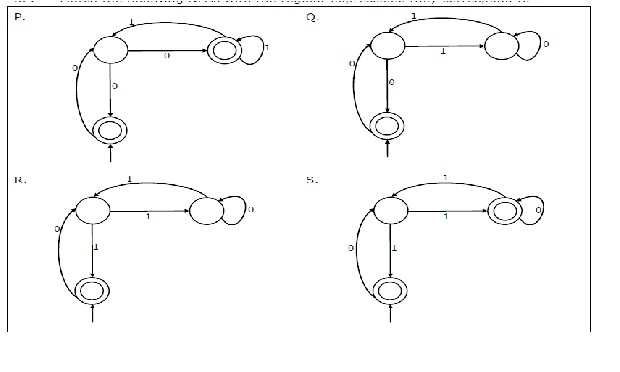(A) P – 2, Q – 1, R – 3, S – 4
(B) P – 1, Q – 3, R – 2, S – 4
(C) P – 1, Q – 2, R – 3, S – 4
(D) P – 3, Q – 2, R – 1, S – 4

Explanation: Trick: Here we see in all the given figures then second state has a loop over the input alphabets. In such cases we should resolve the loop at that state and transform the NFA into a simpler one to get a regular expression for the NFA.
For resolving the loop, first reach the state where loop is to be resolved then draw all loops over that state and all possible movements to move to the final state.

`Figure P: (when loop resolved at middle state)`

Loop at middle state is either by a 00 or a 01*1.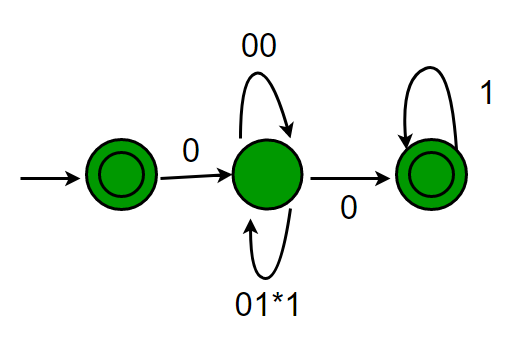Hence the regular expression: € + 0(00 +01*1)* 01*

`Figure Q: (when loop resolved at middle state)`

Loop at middle state is either by a 00 or a 10*1.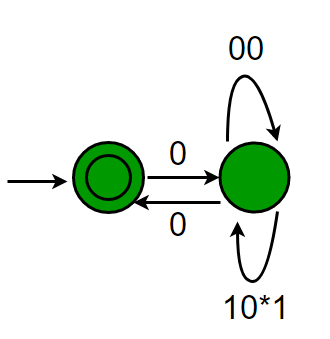Hence the regular expression: € + 0(00 +10*1)* 0.

`Figure R: (when loop resolved at middle state)`

Loop at middle state is either by a 10 or a 10*1.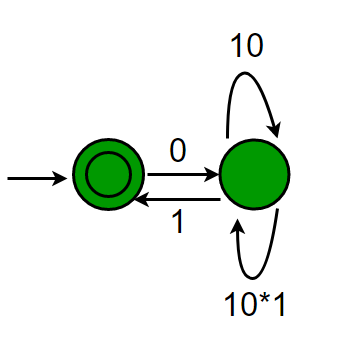Hence the regular expression: € + 0(10 +10*1)* 1.

`Figure S: (when loop resolved at middle state)`

Loop at middle state is either by a 10 or a 10*1.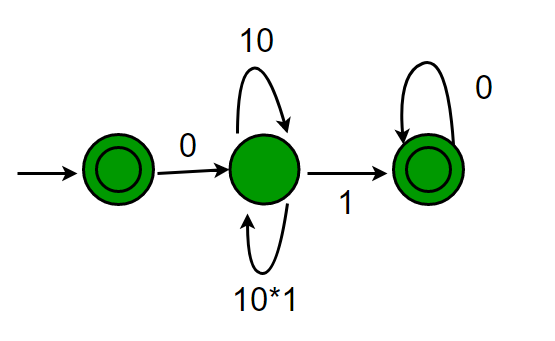Hence the regular expression: € + 0(10 +10*1)* 10*.

The regular expressions matching with the above gives suitable matching as P-1, Q-2, R-3, S-4

This explanation has been contributed by Yashika Arora.

Quiz of this Question

My Personal Notes arrow_drop_up
Article Tags :

1

Please write to us at contribute@geeksforgeeks.org to report any issue with the above content.